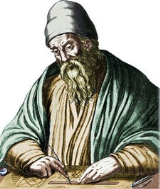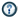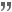xEuclidOverview

Euclid fl.
Floruit
Floruit , abbreviated fl. , is a Latin verb meaning "flourished", denoting the period of time during which something was active...

300 BC, also known as Euclid of Alexandria, was a Greek mathematician
Greek mathematics
Greek mathematics, as that term is used in this article, is the mathematics written in Greek, developed from the 7th century BC to the 4th century AD around the Eastern shores of the Mediterranean. Greek mathematicians lived in cities spread over the entire Eastern Mediterranean, from Italy to...

, often referred to as the "Father of Geometry". He was active in Alexandria
Alexandria
Alexandria is the second-largest city of Egypt, with a population of 4.1 million, extending about along the coast of the Mediterranean Sea in the north central part of the country; it is also the largest city lying directly on the Mediterranean coast. It is Egypt's largest seaport, serving...

during the reign of Ptolemy I (323–283 BC). His Elements
Euclid's Elements
Euclid's Elements is a mathematical and geometric treatise consisting of 13 books written by the Greek mathematician Euclid in Alexandria c. 300 BC. It is a collection of definitions, postulates , propositions , and mathematical proofs of the propositions...

is one of the most influential works in the history of mathematics
History of mathematics
The area of study known as the history of mathematics is primarily an investigation into the origin of discoveries in mathematics and, to a lesser extent, an investigation into the mathematical methods and notation of the past....

, serving as the main textbook
Textbook
A textbook or coursebook is a manual of instruction in any branch of study. Textbooks are produced according to the demands of educational institutions...

for teaching mathematics
Mathematics
Mathematics is the study of quantity, space, structure, and change. Mathematicians seek out patterns and formulate new conjectures. Mathematicians resolve the truth or falsity of conjectures by mathematical proofs, which are arguments sufficient to convince other mathematicians of their validity...

(especially geometry
Geometry
Geometry arose as the field of knowledge dealing with spatial relationships. Geometry was one of the two fields of pre-modern mathematics, the other being the study of numbers ....

) from the time of its publication until the late 19th or early 20th century. In the Elements, Euclid deduced the principles of what is now called Euclidean geometry
Euclidean geometry
Euclidean geometry is a mathematical system attributed to the Alexandrian Greek mathematician Euclid, which he described in his textbook on geometry: the Elements. Euclid's method consists in assuming a small set of intuitively appealing axioms, and deducing many other propositions from these...

from a small set of axiom
Axiom
In traditional logic, an axiom or postulate is a proposition that is not proven or demonstrated but considered either to be self-evident or to define and delimit the realm of analysis. In other words, an axiom is a logical statement that is assumed to be true...

s.DiscussionsWhat are some of his other surviving works?why was Euclids book the "Elements" translated into Arabic and not Latin?What ideas did Euclid put forth in 'The Elements'?Quotations

There is no royal road to geometry.Proclus|Proclus (412–485 AD) in Commentary on the First Book of Euclid's Elements as translated by Glenn R. Morrow (1970)

Give him a coin, since he must profit by what he learns.Attributed by Proclus as a remark about a reluctant student